Documentation

## Modeling Current Signal From an Energizing Transformer

This example shows the modeling of a measured signal. We analyze the current signal from the R-phase when a 400 kV three-phase transformer is energized. The measurements were performed by Sydkraft AB in Sweden.

We describe the use of function `ar` for modeling the current signal. A non-parametric analysis of the signal is first performed. Tools for choosing a reasonable model order are then discussed, along with the use of `ar` for signal modeling. Methods for fitting a model to only a chosen range of harmonics are also discussed.

### Introduction

Signals can be considered as the impulse response of an autoregressive linear model, and can thus be modeled using tools such as `ar`.

Data for signals can be encapsulated into `iddata` objects, by setting the output data of the object to the signal values, and leaving the input empty. For example, if `x(t)` represents a signal to be modeled, then the corresponding `iddata` object can be created as: `data = iddata(x,[],T);`, where `T` is the sample time of `x`.

Standard identification tools, such as `n4sid`, `ssest`, `ar` and `arx` may be used to estimate the characteristics of the "output-only" data. These models are assessed for their spectral estimation capability, as well as their ability to predict the future values of the signal from a measurement of their past values.

### Analyzing Data

We begin this case study by loading the data for the current signal from the transformer:

```load current.mat ```

Now, we package the current data (`i4r`) into an `iddata` object. The sample time is 0.001 seconds (1 `ms`).

```i4r = iddata(i4r,[],0.001) % Second argument empty for no input ```
```i4r = Time domain data set with 601 samples. Sample time: 0.001 seconds Outputs Unit (if specified) y1 ```

Let us now analyze this data. First, take a look at the data:

```plot(i4r) ```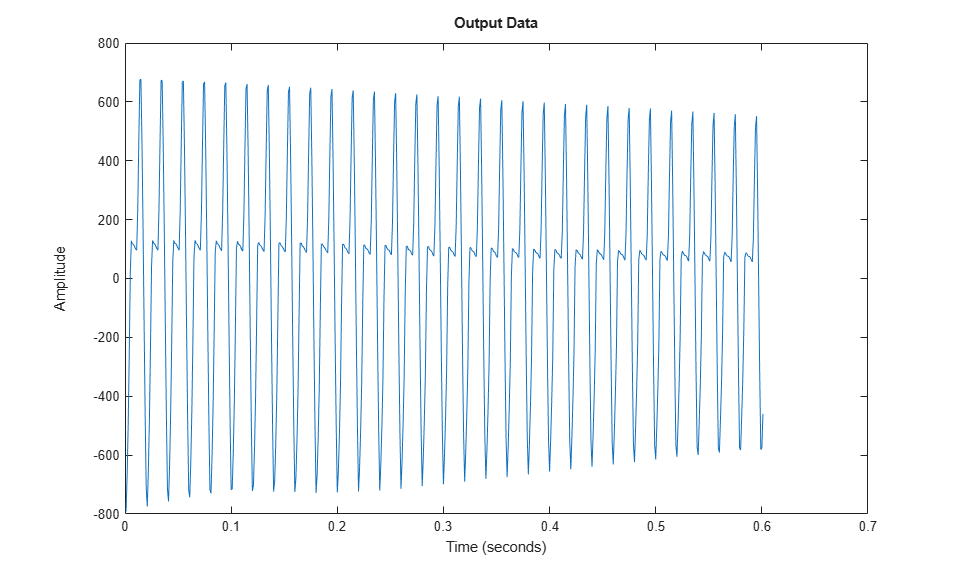A close up view of the data is shown below:

```plot(i4r(201:250)) ```Next, we compute the raw periodogram of the signal:

```ge = etfe(i4r) spectrum(ge) ```
```ge = IDFRD model. Contains spectrum of signal in the "SpectrumData" property. Output channels: 'y1' Status: Estimated using ETFE on time domain data "i4r". ```This periodogram reveals several harmonics, but is not very smooth. A smoothed periodogram is obtained by:

```ges = etfe(i4r,size(i4r,1)/4); spectrum(ge,ges); legend({'ge (no smoothing)','ges (with smoothing)'}) ```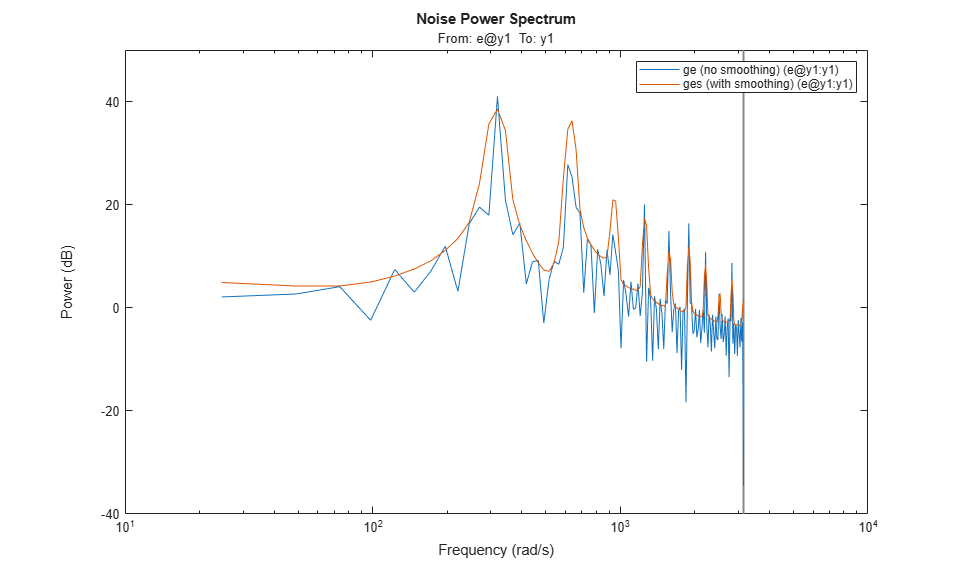Configure the plot to use linear frequency scale and Hz units:

```h = spectrumplot(ges); opt = getoptions(h); opt.FreqScale = 'linear'; opt.FreqUnits = 'Hz'; setoptions(h,opt); axis([0 500,-5 40]) grid on, legend('ges') ```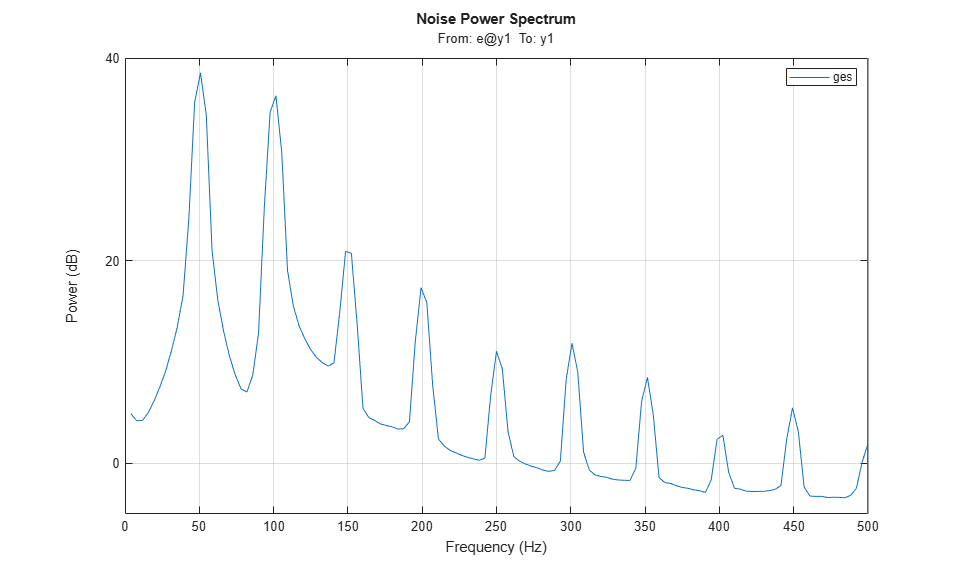We clearly see the dominant frequency component of 50 Hz, and its harmonics.

Let us perform a spectral analysis of the data using `spa`, which uses a Hann window to compute the spectral amplitudes (as opposed to `etfe` which just computes the raw periodogram). The standard estimate (with the default window % size, which is not adjusted to resonant spectra) gives:

```gs = spa(i4r); hold on spectrumplot(gs); legend({'ges (using etfe)','gs (using spa)'}) hold off ```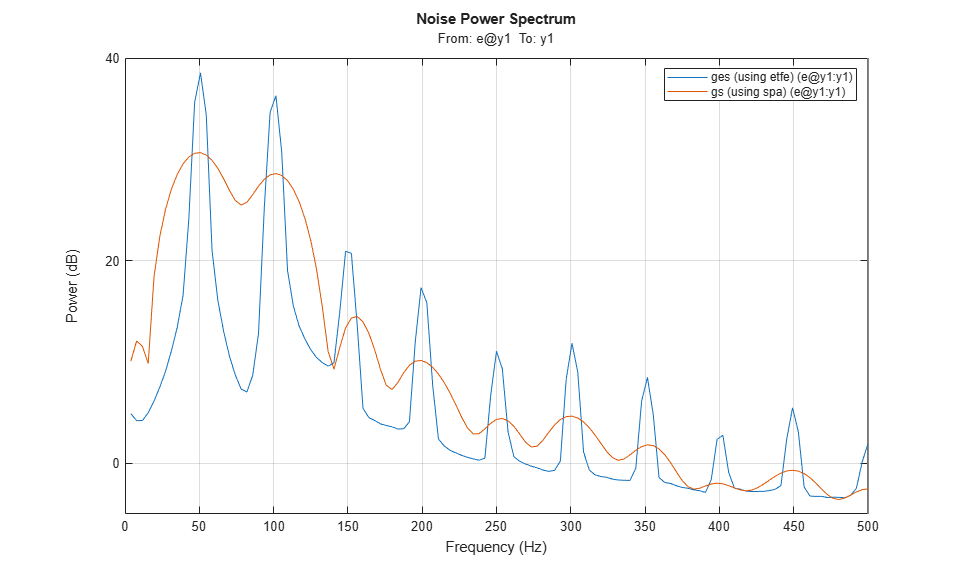We see that a very large lag window will be required to see all the fine resonances of the signal. Standard spectral analysis does not work well. We need a more sophisticated model, such as those provided by parametric autoregressive modeling techniques.

### Parametric Modeling of the Current Signal

Let us now compute the spectra by parametric AR-methods. Models of 2nd 4th and 8th order are obtained by:

```t2 = ar(i4r,2); t4 = ar(i4r,4); t8 = ar(i4r,8); ```

Let us take a look at their spectra:

```spectrumplot(t2,t4,t8,ges,opt); axis([0 500,-8 40]) legend({'t2 (2nd order AR)','t4 (4th order AR)','t8 (8th order AR)','ges (using spa)'}); ```We see that the parametric spectra are not capable of picking up the harmonics. The reason is that the AR-models attach too much attention to the higher frequencies, which are difficult to model. (See Ljung (1999) Example 8.5).

We will have to go to high order models before the harmonics are picked up.

What will a useful order be? We can use `arxstruc` to determine that.

```V = arxstruc(i4r(1:301),i4r(302:601),(1:30)'); % Checking all order up to 30 ```

Execute the following command to select the best order interactively: `nn = selstruc(V,'log');`As the figure above shows, there is a dramatic drop for `n=20`. So let us pick that order for the following discussions.

```t20 = ar(i4r,20); spectrumplot(ges,t20,opt); axis([0 500 -25 80]) legend({'ges (using spa)','t20 (20th order AR)'}); ```All the harmonics are now picked up, but why has the level dropped? The reason is that `t20` contains very thin but high peaks. With the crude grid of frequency points in `t20` we simply don't see the true levels of the peaks. We can illustrate this as follows:

```g20c = idfrd(t20,(551:650)/600*150*2*pi); % A frequency region around 150 Hz spectrumplot(ges,t20,g20c,opt) axis([0 500 -25 80]) legend({'ges (using spa)','t20 (20th order AR)','g20c (resp. around 150 Hz)'}); ```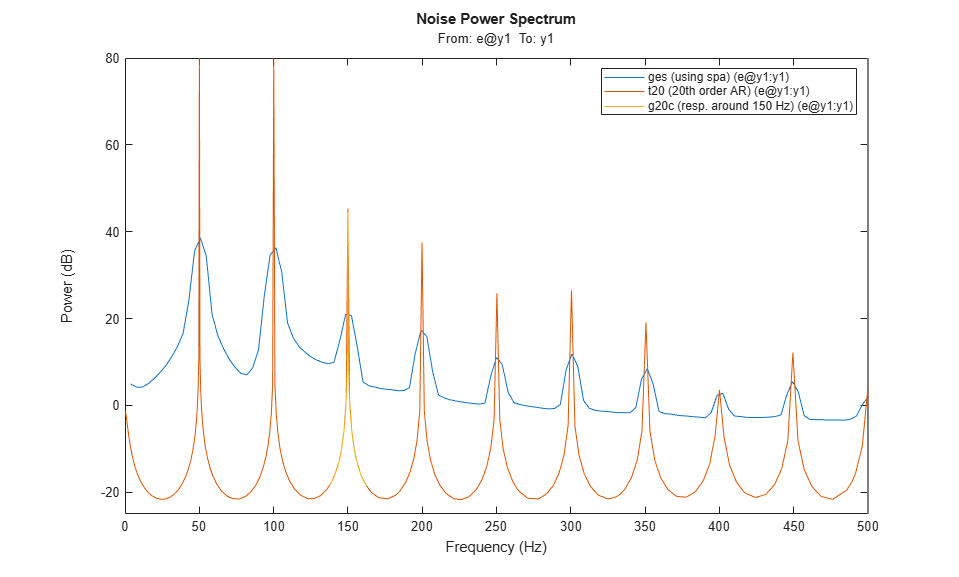As this plot reveals, the model `t20` is fairly accurate; when plotted on a fine frequency grid, it does capture the harmonics of the signal quite accurately.

### Modeling Only the Lower-Order Harmonics

If we are primarily interested in the lower harmonics, and want to use lower order models we will have to apply prefiltering of the data. We select a 5th order Butterworth filter with cut-off frequency at 155 Hz. (This should cover the 50, 100 and 150 Hz modes):

```i4rf = idfilt(i4r,5,155/500); % 500 Hz is the Nyquist frequency t8f = ar(i4rf,8); ```

Let us now compare the spectrum obtained from the filtered data (8th order model) with that for unfiltered data (8th order) and with the periodogram:

```spectrumplot(t8f,t8,ges,opt) axis([0 350 -60 80]) legend({'t8f (8th order AR, filtered data)',... 't8 (8th order AR, unfiltered data)','ges (using spa)'}); ```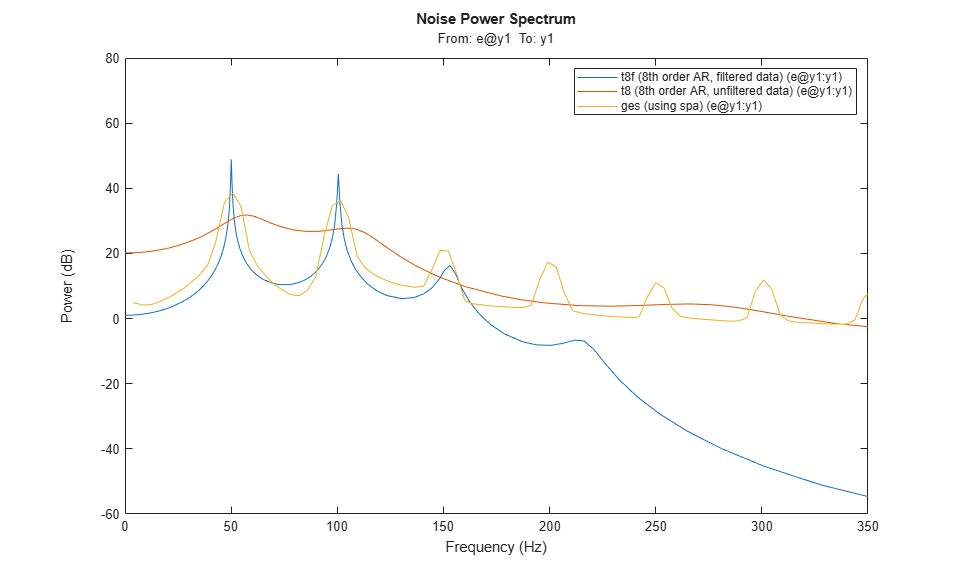We see that with the filtered data we pick up the first three peaks in the spectrum quite well.

We can compute the numerical values of the resonances as follows: The roots of a sampled sinusoid of frequency, say `om`, are located on the unit circle at `exp(i*om*T)`, `T` being the sample time. We thus proceed as follows:

```a = t8f.a % The AR-polynomial omT = angle(roots(a))' freqs = omT/0.001/2/pi'; % show only the positive frequencies for clarity: freqs1 = freqs(freqs>0) % In Hz ```
```a = Columns 1 through 7 1.0000 -5.0312 12.7031 -20.6934 23.7632 -19.6987 11.5651 Columns 8 through 9 -4.4222 0.8619 omT = Columns 1 through 7 1.3591 -1.3591 0.9620 -0.9620 0.3146 -0.3146 0.6314 Column 8 -0.6314 freqs1 = 216.3063 153.1036 50.0665 100.4967 ```

We thus find the first three harmonics (50, 100 and 150 Hz) quite well.

We could also test how well the model `t8f` is capable of predicting the signal, say 100 ms (100 steps) ahead, and evaluate the fit on samples 201 to 500:

```compare(i4rf,t8f,100,compareOptions('Samples',201:500)); ```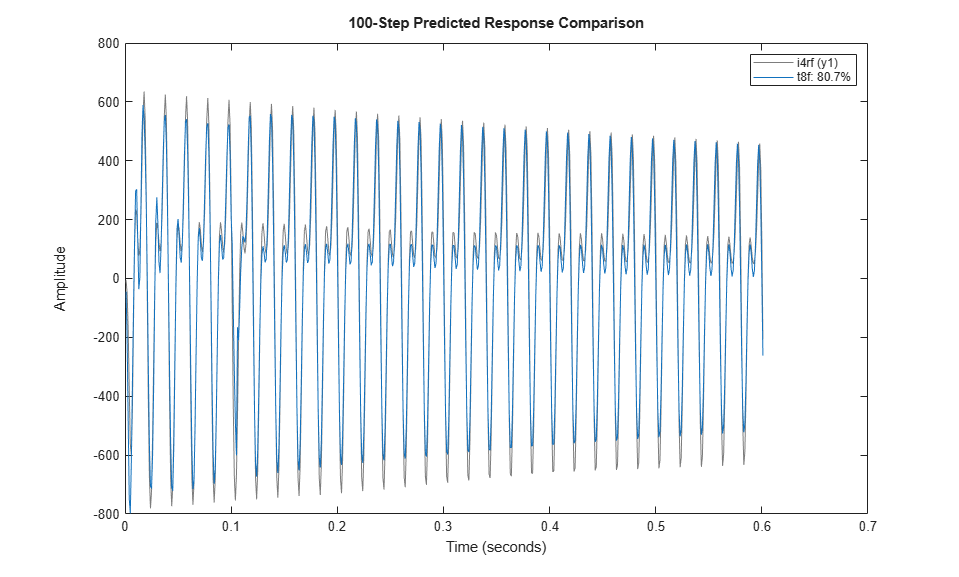As observed, a model of the first 3 harmonics is pretty good at predicting the future output values, even 100 steps ahead.

### Modeling Only the Higher-Order Harmonics

If we were interested in only the fourth and fifth harmonics (around 200 and 250 Hz) we would proceed by band-filtering the data to this higher frequency range:

```i4rff = idfilt(i4r,5,[185 275]/500); t8fhigh = ar(i4rff,8); spectrumplot(ges,t8fhigh,opt) axis([0 500 -60 40]) legend({'ges (using spa)','t8fhigh (8th order AR, filtered to high freq. range)'}); ```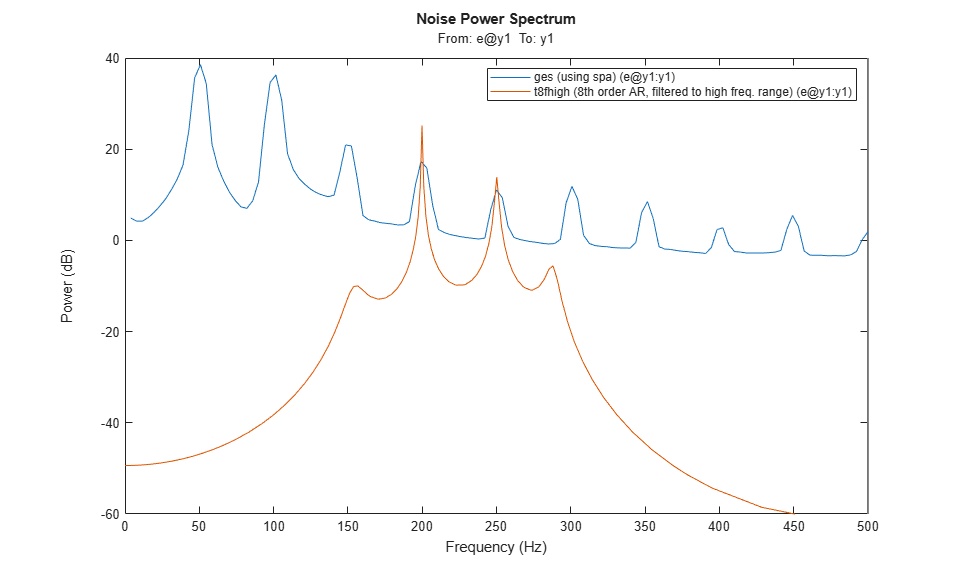We thus got a good model in `t8fhigh` for describing the 4th and 5th harmonics. We thus see that with proper prefiltering, low order parametric models can be built that give good descriptions of the signal over the desired frequency ranges.

### Conclusions

Which model is the best? In general, a higher order model would give a higher fidelity. To analyze this, we consider what the 20th order model would give in terms of its capability in estimating harmonics:

```a = t20.a % The AR-polynomial omT = angle(roots(a))' freqs = omT/0.001/2/pi'; % show only the positive frequencies for clarity: freqs1 = freqs(freqs>0) %In Hz ```
```a = Columns 1 through 7 1.0000 0.0034 0.0132 0.0012 0.0252 0.0059 0.0095 Columns 8 through 14 0.0038 0.0166 0.0026 0.0197 -0.0013 0.0143 0.0145 Columns 15 through 21 0.0021 0.0241 -0.0119 0.0150 0.0246 -0.0221 -0.9663 omT = Columns 1 through 7 0 0.3146 -0.3146 0.6290 -0.6290 0.9425 -0.9425 Columns 8 through 14 1.2559 -1.2559 1.5726 -1.5726 1.8879 -1.8879 2.2027 Columns 15 through 20 -2.2027 2.5136 -2.5136 3.1416 2.8240 -2.8240 freqs1 = Columns 1 through 7 50.0639 100.1139 149.9964 199.8891 250.2858 300.4738 350.5739 Columns 8 through 10 400.0586 500.0000 449.4611 ```

We see that this model picks up the harmonics very well. This model will predict 100 steps ahead as follows:

```compare(i4r,t20,100,compareOptions('Samples',201:500)); ```We now have a 93% fit with `t20`, as opposed to 80% for `t8f`.

We thus conclude that for a complete model of the signal, `t20` is the natural choice, both in terms of capturing the harmonics as well as in its prediction capabilities. For models in certain frequency ranges we can however do very well with lower order models, but we then have to prefilter the data accordingly.